# 【Flutter 绘制技巧】Path 路径变换#### 1. 绘制路径测试

``````void main() {
runApp(CustomPaint(
painter: PathPainter(),
));
}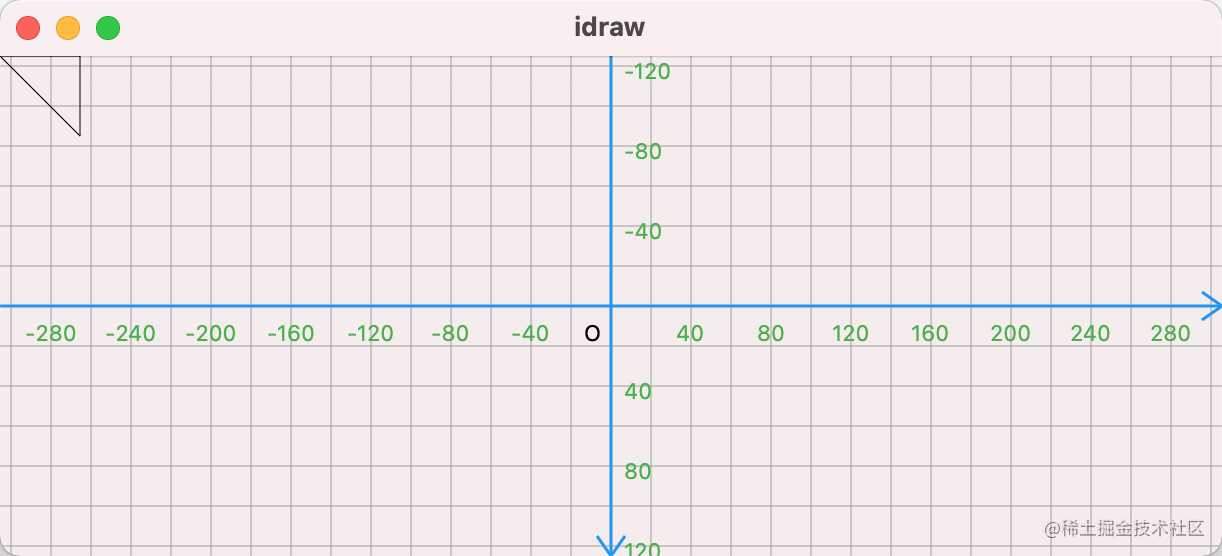``````class PathPainter extends CustomPainter {

@override
void paint(Canvas canvas, Size size) {
Paint paint = Paint()..style = PaintingStyle.stroke;
Path path = Path()
..lineTo(40, 40)
..relativeLineTo(0, -40)
..close();
canvas.drawPath(path, paint);
}

@override
bool shouldRepaint(covariant PathPainter oldDelegate) => true;
}

#### 2.画板的变换和路径的变换

``````---->[extra_03_path/01]----
canvas.translate(size.width/2, size.height/2,);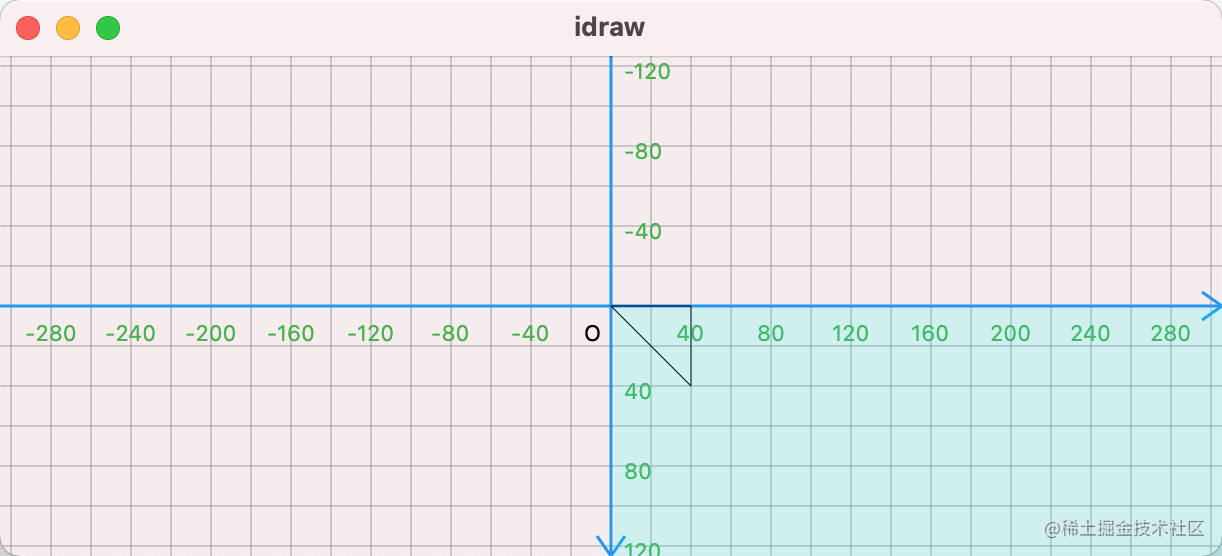``````---->[extra_03_path/02]----
Path path = Path()
..moveTo(size.width/2, size.height/2,)
..relativeLineTo(40, 40)
..relativeLineTo(0, -40)
..close();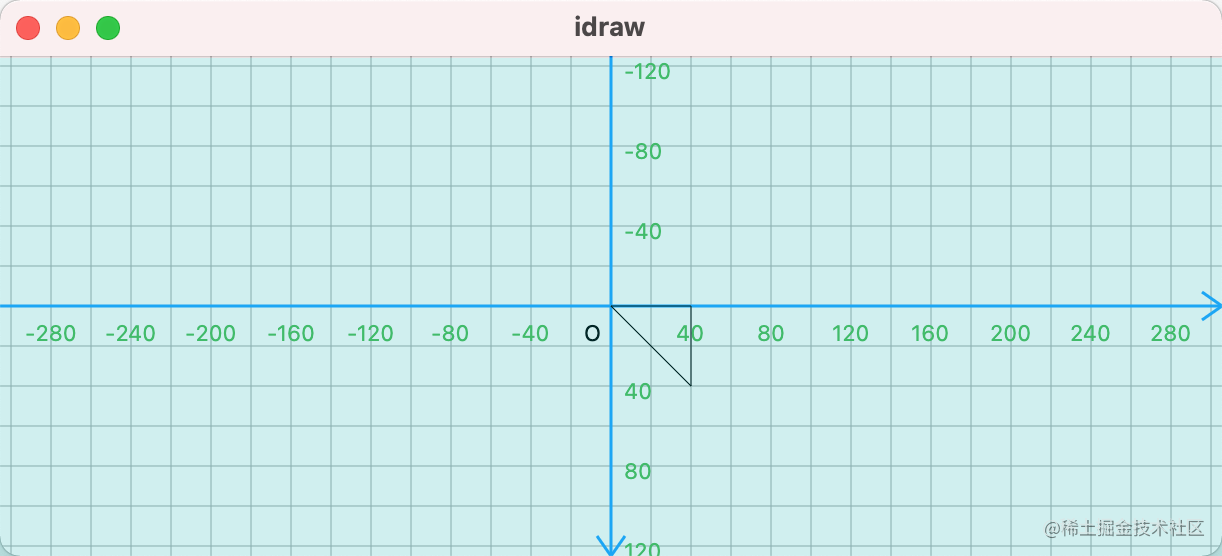``````---->[extra_03_path/03]----
print(path.contains(Offset(30,10))); //true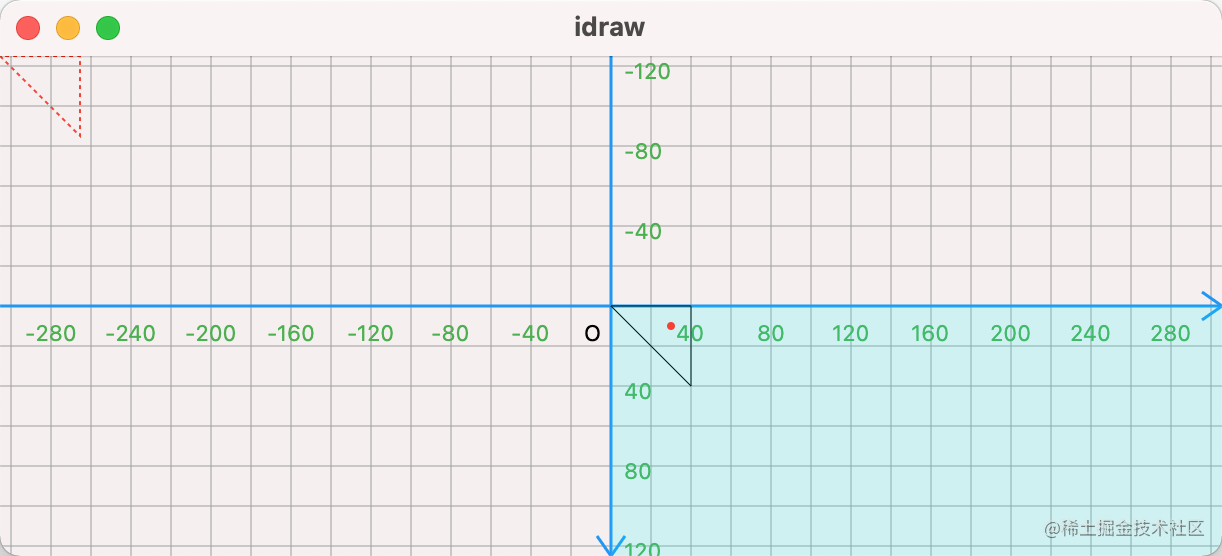#### 3. 路径变换

``````---->[extra_03_path/05]----
Matrix4 m4 = Matrix4.rotationZ(10*pi/180);
path = path.transform(m4.storage);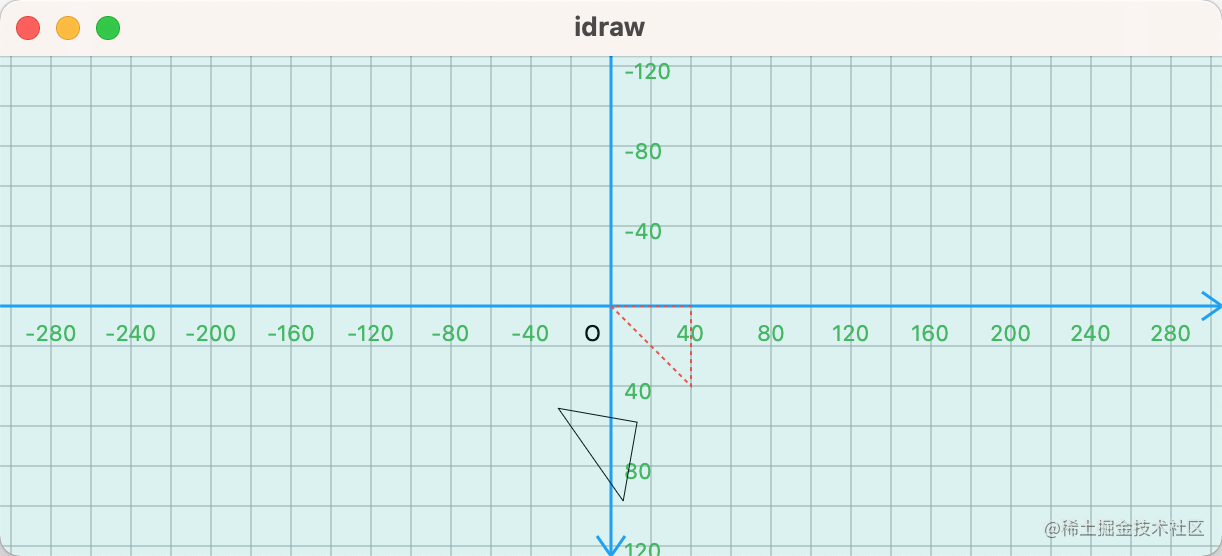那如何指定某点为变换中心呢？在一次变换中，通过平移，可以改变变换中心。比如下面左上角的红色虚线路径，通过 `平移变换` ，形成如下黑线路径。

``````---->[extra_03_path/06]----
Path path = Path()
..moveTo(0, 0)
..relativeLineTo(40, 40)
..relativeLineTo(0, -40)
..close();

Matrix4 m4 = Matrix4.translationValues(size.width/2, size.height/2, 0);
path = path.transform(m4.storage);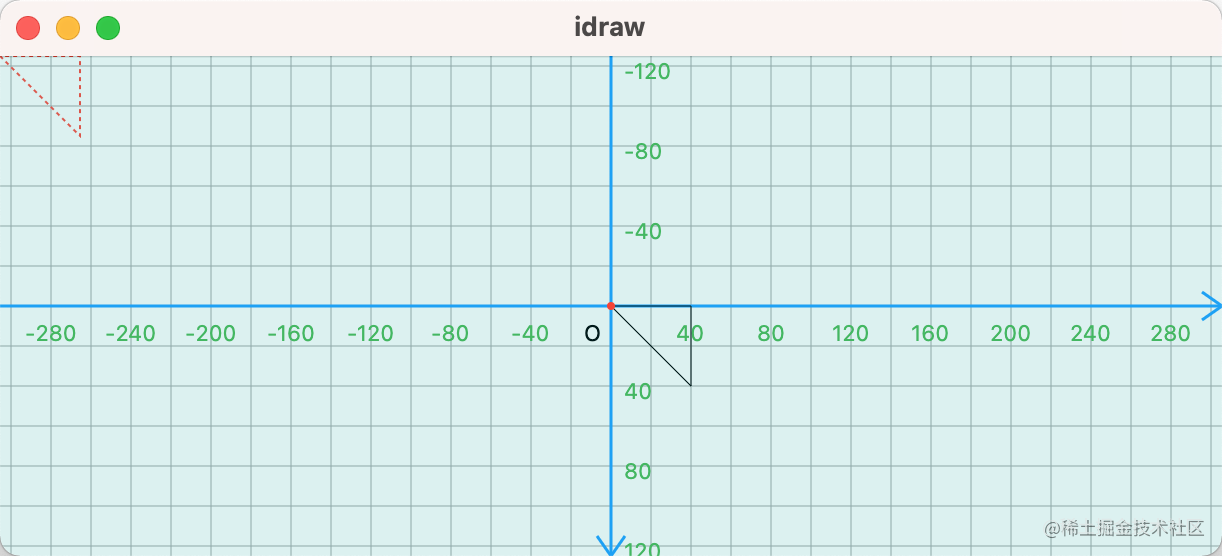``````---->[extra_03_path/07]----
Matrix4 m4 = Matrix4.translationValues(size.width/2, size.height/2, 0);
Matrix4 rotateM4 = Matrix4.rotationZ(10*pi/180);
m4.multiply(rotateM4);
path = path.transform(m4.storage);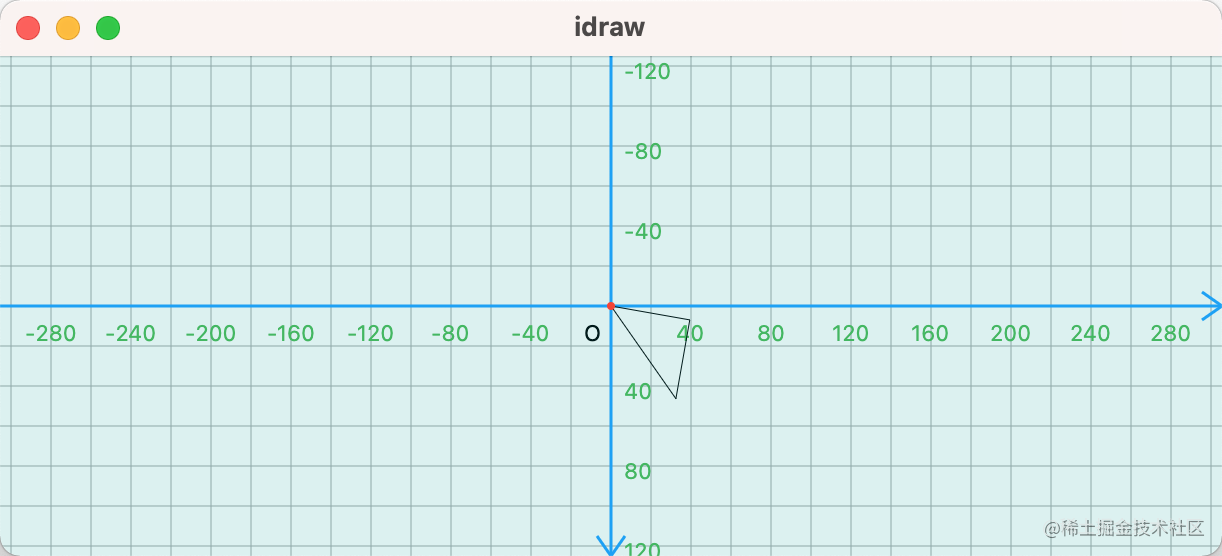``````---->[extra_03_path/08]----
Matrix4 m4 = Matrix4.translationValues(size.width/2, size.height/2, 0);
Matrix4 rotateM4 = Matrix4.rotationZ(10*pi/180);
Matrix4 scaleM4 = Matrix4.diagonal3Values(2,2,1);
m4.multiply(rotateM4);
m4.multiply(scaleM4);
path = path.transform(m4.storage);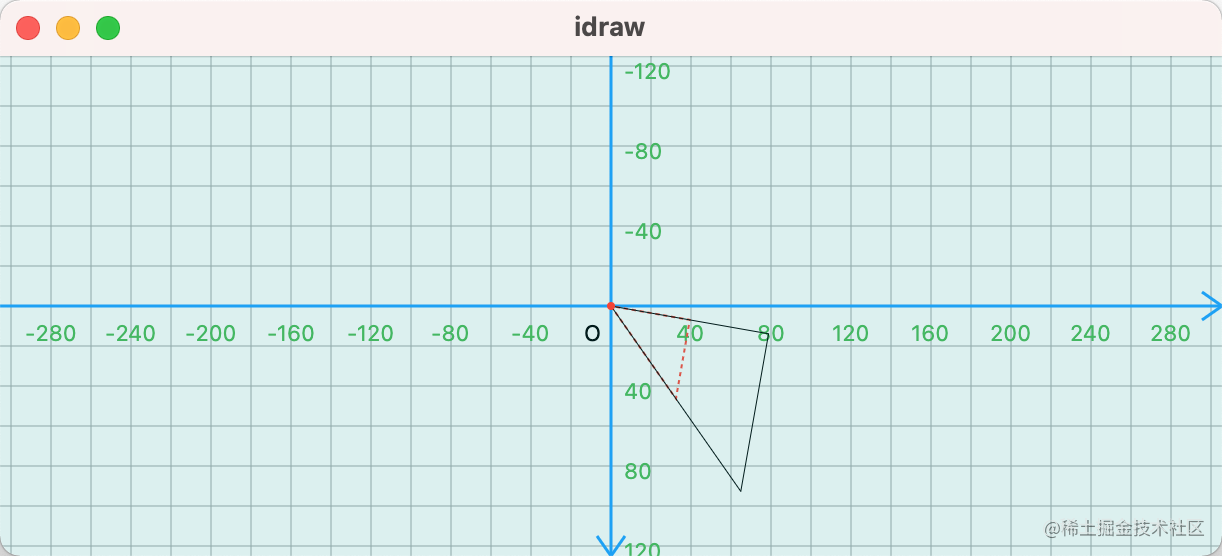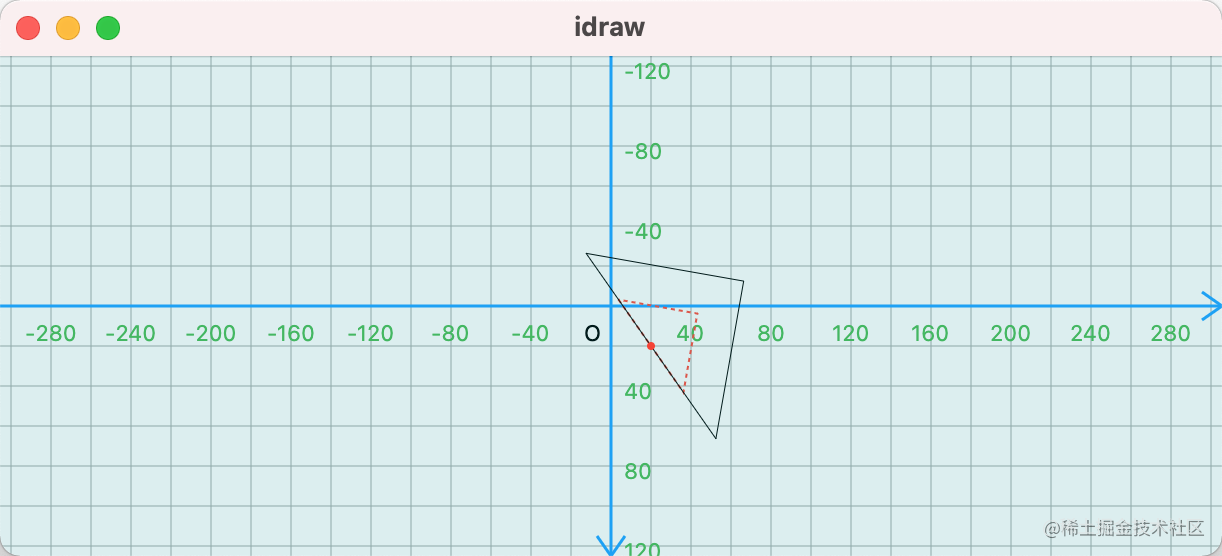``````---->[extra_03_path/09]----
Matrix4 m4 = Matrix4.translationValues(size.width/2, size.height/2, 0);
Matrix4 center = Matrix4.translationValues(20, 20, 0);
Matrix4 back = Matrix4.translationValues(-20, -20, 0);

Matrix4 rotateM4 = Matrix4.rotationZ(10*pi/180);
Matrix4 scaleM4 = Matrix4.diagonal3Values(2,2,1);

m4.multiply(center);// tag1
m4.multiply(rotateM4);
m4.multiply(scaleM4);
m4.multiply(back); // tag2
path = path.transform(m4.storage);
canvas.drawPath(path, paint);

#### 4. 路径变换与命中

``````---->[extra_03_path/10]----
bool contains = path.contains(pos.value);
double strokeWidth = contains ? 2 : 1;
Color color = contains ? Colors.orange : Colors.black;
canvas.drawPath(path, paint..strokeWidth = strokeWidth..color = color);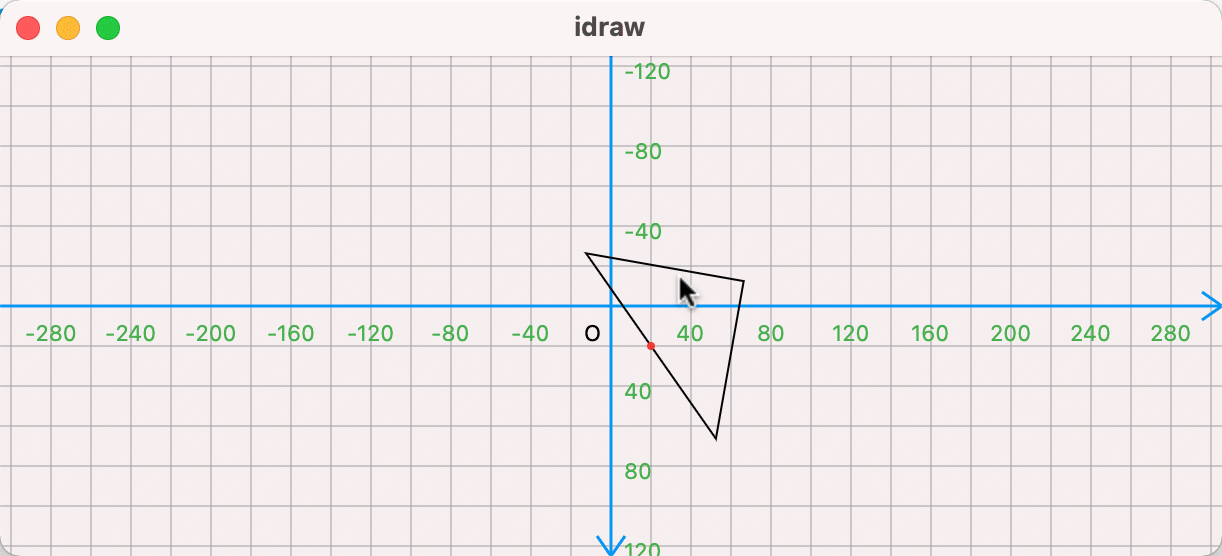`multiply` 方法，如下所示：是根据矩阵的乘法，来修改自身的数据。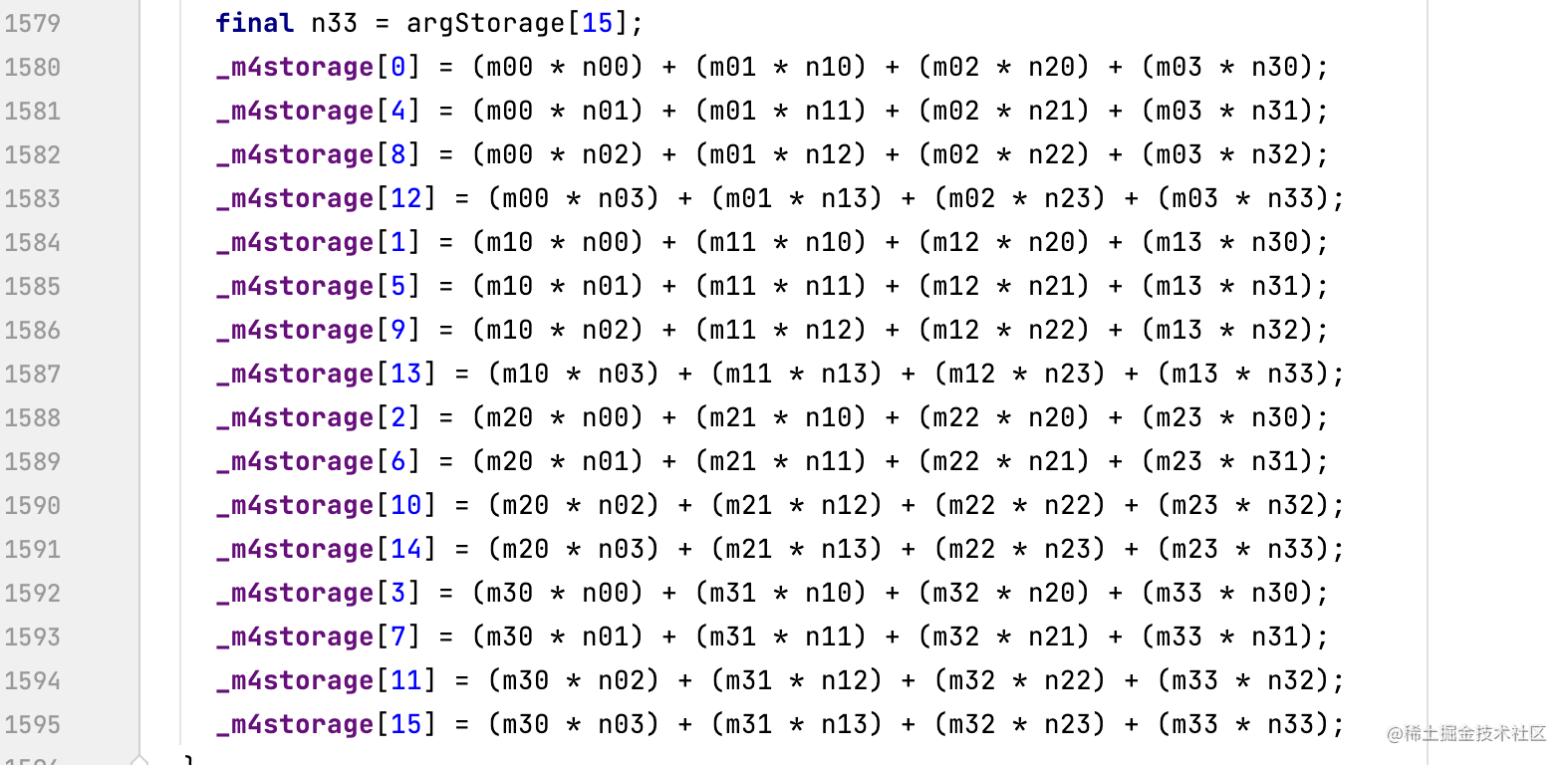Android

Android